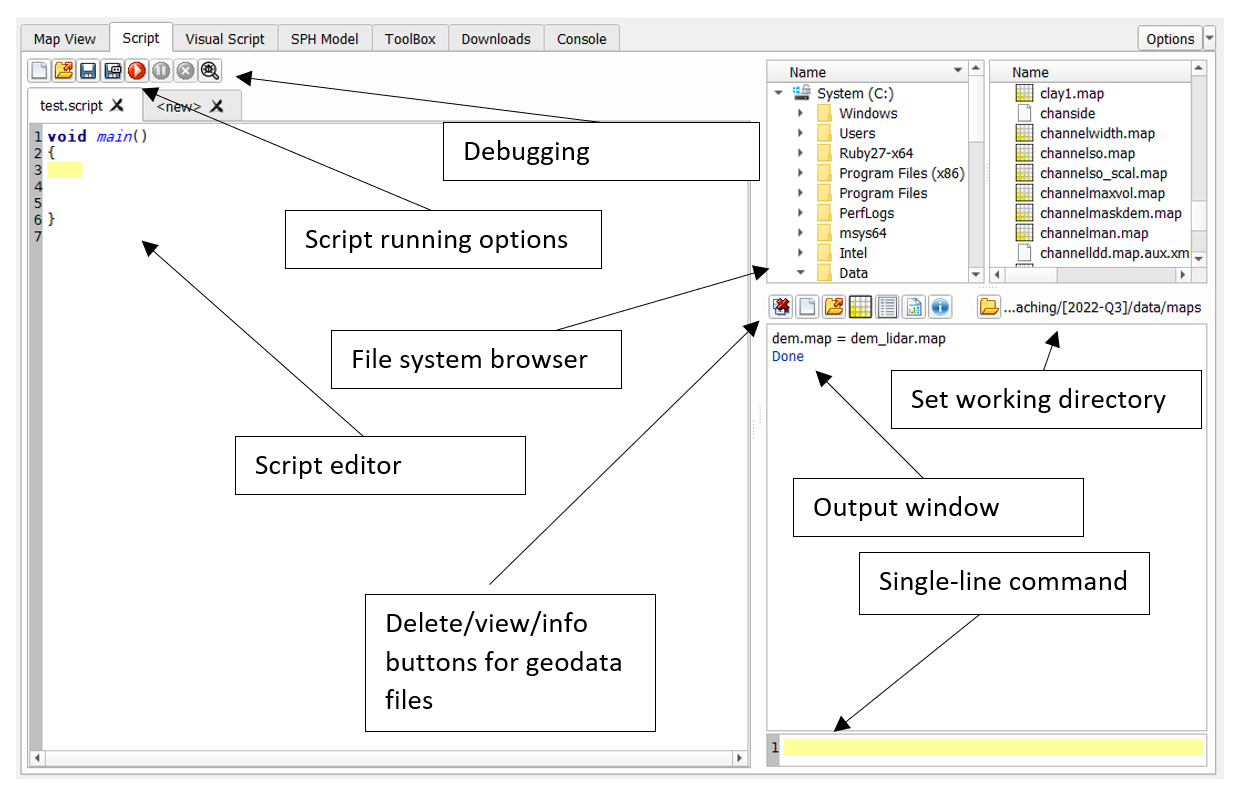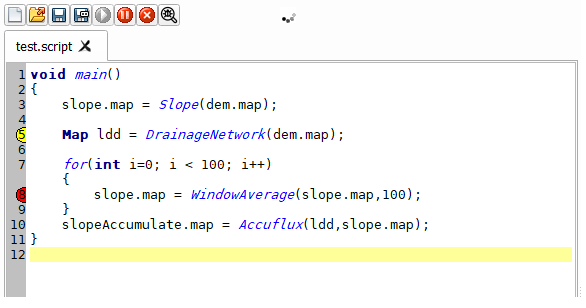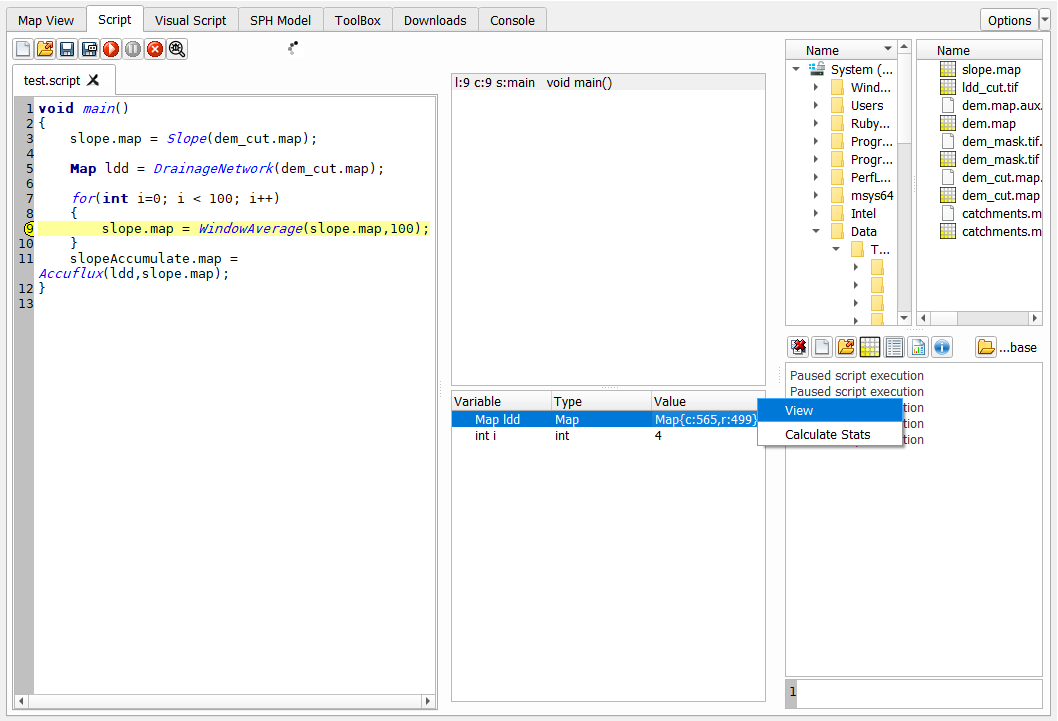LISEM Classic

LISEM

# Scripting

The scripting environment not only provides a command-line, but also features a full script editor.The script editor provides a more full scripting environment based on a customized version of the angelscript languge. The angelscript language is a compiled, statically-types c-style language that features various advanced language features. Provided functions link directly to compiled c++ code without performence loss, and often utilizing all available cores. Here, the in-line references to files are still valid, but can be avoided if desired. A script always starts with a void main() function. This function is the entry point, and if it is not found, the script can not be run. A simple script might combine map calculations and avoid nearly all of the technicalities of the scripting language:

void main()
{
a.tif = b.tif + 2.0;
slope.tif = Slope(dem.tif);
angle.tif = Sin(Atan(Slope(dem.tif)));

a.map = MapMaximum(angle.tif)
}


Files in such a script are found in the selected working directory. However, the scripting langue features many more functionalities, as demonstrated below:

//this is a comment
//here the main() function is defined
//the entry point
void main()
{
a.map = b.map;
Map a = a.map;

//Load… functions take a path relative to the working directory
Map b = LoadMap(“b.map”)

//The Load.. functions have a variant that takes an absolute path
Shapes s = LoadShapesAbsPath(“C:/s.shp”)

//this loop runs for 100 iterations
for(int i = 0; i < 100; i++)
{
}
}

//define another function call AddToB, it takes 1 argument of type Map
{
b += c.map;
return b;
}


### Data types

There are various basic data types available:

1. bool
2. int
3. uint
4. float
5. double
6. string
7. int and unsigned int types of various sizes (int16, uint16, etc..)

Besides these, several geospatial datatypes are defined by LISEM

1. Map
2. Shapes
3. PointCloud
4. Object
5. Table
6. GeoProjection
7. MathExpression

Linear algebra types are available based on common names in computer graphics.

1. vector types vec2, vec3 and vec4
2. matrix types mat2x2, mat3x3 and mat4x4.

Text can be represented with the string type. Quotations between brackets (“…”) are automatically of type string. Basic datatypes can be converted to string using the ToString() function. Strings can be manipulated just as other types by using the operators. (+ to join strings).

Lists of data can be defined and used with the Array type. You can load a list of bands from a geotiff file.

Array<Map> bands = LoadMapBandList(spectral.tif)


Array is a templated type. This means that there is a special template argument between angled brackets (<..>). This is the type that is stored in the list. You can make lists of any type (Array<Array> can represent a matrix of text).

### Operators

Most of the datatypes, both basic, geospatial and otherwise, support manipulation through operators. Supported operators are + (addition),- (substraction),/ (division),* (multiplication),** (power),% (modulus),< (less than),> (greater than),== (equal to),» (right shift),« (left shift),& (and),| (or), ^ (xor), [] (list indexing). Because of some limitations of the language, the <, > and == operators can not be overloaded. Instead, the non-basic types support «, » and .eq as the less than, greater than and equal to operators. So, for rasters:

Conditional.tif = MapIf( (a.map >> b.map) & (c.tif.eq(d.tif)), iftrue.tif, iffalse.tif)


### Conditional

There is support for if/else statements

void main()
{
if(true)
{
Print("This is printed")
}else
{
Print("This is not printed")
}
}


### Functions

Functions follow a c-style format. Of course, when the return type is void (nothing), no return statement is required.

returntype FunctionName(argumenttype argumentname)
{
returntype returnvariable;

//calculations
return returnvariable;
}


or, as example

int fibonacci(int n)
{
if(n <= 1)
{
return n;
}else
{
return fibonacci(n-1) + fibonacci(n-2);
}
}


### Classes

Classes can be defined using a c-style syntax. Inheritence is possible, allowing for base classes or interfaces. Classes can have member functions and member variables, which are unique for each instance of that class.

// The class declaration
class MyClass
{
// A class method
void DoSomething()
{
// The class properties can be accessed directly
a *= 2;
// The declaration of a local variable may hide class properties
int b = 42;
// In this case the class property have to be accessed explicitly
this.b = b;
}
// Class properties
int a;
int b;
}


### Templates

Templated types are class objects that take a template parameter to determine the full type. For example, the array type is templated, meaning that it is not possible to have a simple array. Instead, the type of the object contained within must be specified: array<double> represents a list of numbers. This can be nested as: array<array<double>>. This would represent a matrix of numbers.

### Debugging

A simple debugger and stack view is present in the script tool. By clicking on a line number, a breakpoint can be added. Once the script encounters a breakpoint, it will be paused, and you can inspect all the variables currently in memory.The debug window can be openend and closed using the debug button on the top. The top window shows that stack. This is a list of all the nested function calls. In the image, the stack is 1 level deep, as we are in main(), and no further function call has been made. The variables are shown, with a short description. A copy of Map objects can be added to the map viewer, or the statistics (size, average, minimum, maximum and standard deviation) can be shown.### File shortcut support

Angelscript does not natively support the usage of files as variables in a script. The extension here works by automatically finding occurrences of files within the script by looking for file extensions. Common file extensions for rasters, vector files, tables and 3d models are supported. If such a file exists in your current working directory, the script is changed to call the appropriate function. So, for slope.map = Slope(dem.map), the script is converted to: void main() { SaveThisMapAs(“slope.map”) = Slope(LoadMap(“dem.map”)); } Where SaveThisMapAs, returns a special object that is written to file upon assignment.